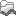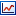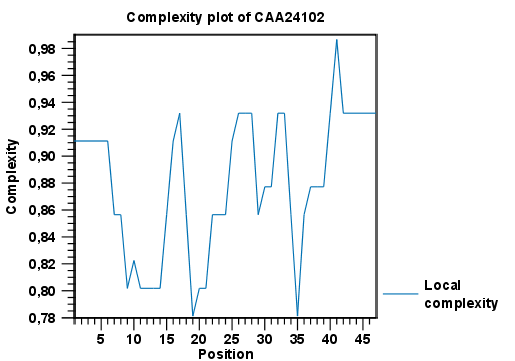Local complexity plot

In CLC Genomics Workbench it is possible to calculate local complexity for both DNA and protein sequences. The local complexity is a measure of the diversity in the composition of amino acids within a given range (window) of the sequence. The K2 algorithm is used for calculating local complexity [Wootton and Federhen, 1993]. To conduct a complexity calculation do the following:

Select sequences in Navigation Area | Toolbox in Menu Bar | Classical Sequence Analysis () | General Sequence Analysis ()| Create Complexity Plot ()

This opens a dialog. In Step 1 you can change, remove and add DNA and protein sequences.

When the relevant sequences are selected, clicking Next takes you to Step 2. This step allows you to adjust the window size from which the complexity plot is calculated. Default is set to 11 amino acids and the number should always be odd. The higher the number, the less volatile the graph.

Figure 14.14 shows an example of a local complexity plot.Figure 14.14: An example of a local complexity plot.

Click Next if you wish to adjust how to handle the results. If not, click Finish. The values of the complexity plot approaches 1.0 as the distribution of amino acids become more complex.

See Graph preferences for information about the graph view.